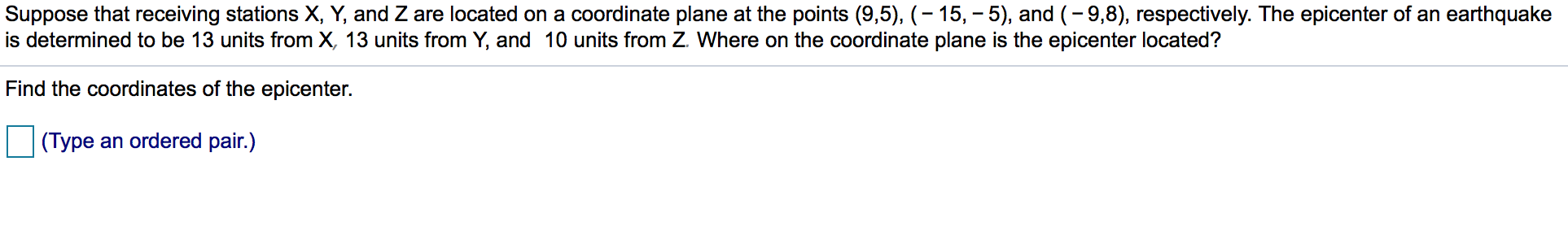Suppose that receiving stations X, Y, and Z are located on a coordinate plane at the points (9,5), -15, -5), and (-9,8), respectively. The epicenter of an earthquakeis determined to be 13 units from X, 13 units from Y, and 10 units from Z Where on the coordinate plane is the epicenter located?Find the coordinates of the epicenter.an ordered pair.)(Type

Questionhelp_outlineImage TranscriptioncloseSuppose that receiving stations X, Y, and Z are located on a coordinate plane at the points (9,5), -15, -5), and (-9,8), respectively. The epicenter of an earthquake is determined to be 13 units from X, 13 units from Y, and 10 units from Z Where on the coordinate plane is the epicenter located? Find the coordinates of the epicenter. an ordered pair.) (Type fullscreen
Step 1

Consider the receiving stations:

Step 2

Now, let the coordinates of epicenter are (x, y),

Since, epicenter is 13 units from X, 13 units from Y, and 10 units from Z.

So, by distance formula between two points:

We will have,

Step 3

Thus, we will get the fol...

Want to see the full answer?

See Solution

Want to see this answer and more?

Our solutions are written by experts, many with advanced degrees, and available 24/7

See Solution
Tagged in

Algebra